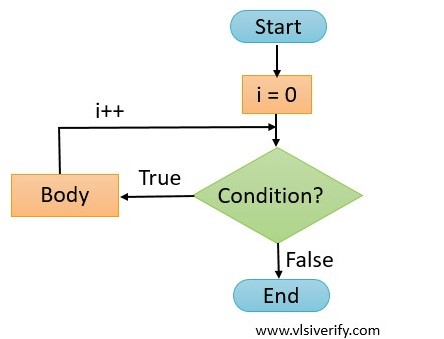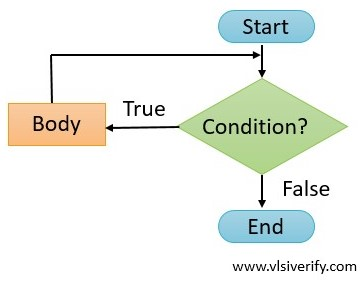Tutorials

# Loops in Verilog

A loop is an essential concept of any programming language. The loop is useful to read/ update an array content, execute a few statements multiple times based on a certain condition. All looping statements can only be written inside procedural (initial and always) blocks. In Verilog, we will discuss the following loop blocks.

1. For loop
2. While loop
3. Forever loop
4. Repeat loop

In all supported loops, begin and end keywords are used to enclose multiple statements as a single block. A begin and end keywords are optional if the loop encloses a single statement.

For loop

The for loop iterates till the mentioned condition is satisfied. The execution of for loop depends on –

1. Initialization
2. Condition
3. Update

Syntax:

``````for (<initialization>; <condition>; <update>) begin
...
end``````
1. Initialization: An initial value of the variable is set. It is executed only once.
2. Condition: A condition or expression is evaluated. If it is evaluated to be the true body of for loop (statements inside begin and end) are executed else, the loop terminates.
3. Update: After execution of for loop body, the variable value is updatedfor loop flow chart

Example:

``````module for_example;
int array;

initial begin
// Update array
for (int i = 0; i < \$size(array); i++) begin
array[i] = i*i;
end

// Display array elements
for (int i = 0; i < \$size(array); i++) begin
\$display("array[%0d] = %0d", i, array[i]);
end
end
endmodule``````

Output:

``````array = 0
array = 1
array = 4
array = 9
array = 16
array = 25
array = 36
array = 49
array = 64
array = 81``````

It is possible to have multiple variable initializations, conditions, and update values.

``````module for_example;
int array;

initial begin
// Update array
for (int i = 0, cnt = 0; i < \$size(array); i++, cnt++) begin
array[i] = i*i;
\$display("cnt = %0d", cnt);
end

// Display array elements
for (int i = 0; i < \$size(array); i++) begin
\$display("array[%0d] = %0d", i, array[i]);
end
end
endmodule``````

Output:

``````cnt = 0
cnt = 1
cnt = 2
cnt = 3
cnt = 4
cnt = 5
cnt = 6
cnt = 7
cnt = 8
cnt = 9
array = 0
array = 1
array = 4
array = 9
array = 16
array = 25
array = 36
array = 49
array = 64
array = 81``````

A for loop behaves as a while loop if initializations, conditions, and update values are not written.

``````module for_example;
int cnt;
initial begin
for (;;) begin
\$display("cnt = %0d", cnt);
if(cnt == 10) break;
cnt++;
end
end
endmodule``````

Output:

``````cnt = 0
cnt = 1
cnt = 2
cnt = 3
cnt = 4
cnt = 5
cnt = 6
cnt = 7
cnt = 8
cnt = 9
cnt = 10``````

## While loop

A while loop is a control flow statement that executes statements repeatedly if the condition holds true else loop terminates

Syntax:

``````while(<condition>) begin
...
end ``````while loop flow chart

Example:

In the below example, the value of ‘count’ is incremented till the condition holds true.

``````module while_example;
int count;
initial begin
while(count<10) begin
\$display("Value of count = %0d", count);
count++;
end
end
endmodule``````

Output:

``````Value of count = 0
Value of count = 1
Value of count = 2
Value of count = 3
Value of count = 4
Value of count = 5
Value of count = 6
Value of count = 7
Value of count = 8
Value of count = 9``````

Note: The ‘do while’ loop is not supported in Verilog.

## Forever loop

As the name suggests, a forever loop runs indefinitely. To terminate the loop, a ‘disable’ statement can be used. The forever loop is the same as the while loop with an expression that holds always true i.e. while(1) loop.

The forever loop must be used with a timing control construct otherwise the simulator will get stuck in a zero-delay simulation time loop.

Syntax:

``````forever begin
...
end``````

Example with \$finish:

To terminate the loop, \$finish system call is used.

``````module forever_example;
integer count = 0;
initial begin
forever begin
\$display("Value of count = %0d", count);
count++;
#5;
end
end

initial begin
#30;
\$finish;
end
endmodule``````
``````Value of count = 0
Value of count = 1
Value of count = 2
Value of count = 3
Value of count = 4
Value of count = 5
\$finish called from file "testbench.sv", line 16.
\$finish at simulation time 30``````

Note: The break and disable statements are not synthesizable in Verilog

### Difference between always and forever block

Both always and forever block the same effect. The always block is a procedural block and it can not be placed inside other procedural blocks.

Syntax: always

``````// For multiple statements in always block
always begin
...
end

// For single statement
always <single statement>
``````

Example of always block:

``````module always_example;
int count;
always begin
\$display("Value of count = %0d", count);
count++;
#5;
end

initial begin
#30;
\$finish;
end
endmodule``````

Output:

``````Value of count = 0
Value of count = 1
Value of count = 2
Value of count = 3
Value of count = 4
Value of count = 5
\$finish called from file "testbench.sv", line 14.
\$finish at simulation time 30``````

#### A always block inside another procedural block

A compilation error is expected when always block is used inside another procedural block i.e. initial begin .. end block. In such a case, a forever block can be used.

``````module always_example;
int count;
initial begin
always begin // can not use inside other procedural block
\$display("Value of count = %0d", count);
count++;
#5;
end
end

initial begin
#30;
\$finish;
end
endmodule``````

Output:

``````Error-[SE] Syntax error
Following verilog source has syntax error :
"testbench.sv", 7: token is 'always'
always begin // can not use inside other procedural block
^

1 error
CPU time: 1.120 seconds to compile
Exit code expected: 0, received: 1``````

## Repeat loop

A repeat loop is used to execute statements a given number of times.

Syntax:

``````repeat(<number>) begin   // <number> can be variable or fixed value
...
end``````

Example:

In the below example,

1. Based on the array size, array elements are printed.
2. The string “Repeat it” is printed three times.
``````module repeat_example;
int array = '{100, 200, 300, 400, 500};
int i;
initial begin
repeat (\$size(array)) begin
\$display("array[%0d] = %0d", i, array[i]);
i++;
end

repeat(3)
\$display("Repeat it");
end
endmodule``````

Output:

``````array = 100
array = 200
array = 300
array = 400
array = 500
Repeat it
Repeat it
Repeat it``````

Verilog Tutorials